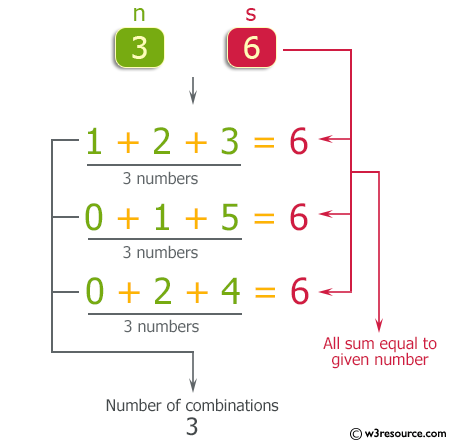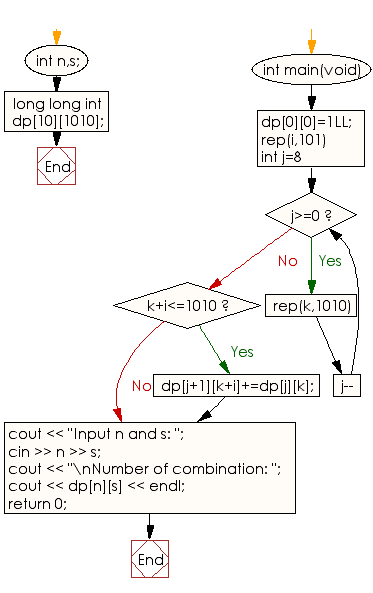﻿ C++ : Accepts n and s which is equal to the sum of the n

# C++ Exercises: Accepts n different numbers and s which is equal to the sum of the n different numbers

## C++ Basic: Exercise-80 with Solution

Write a C++ program that accepts n different numbers (0 to 100) as well as an integer s which is equal to the sum of the n different numbers.
Your job is to find the number of combinations of n numbers and the same number cannot be used for one combination

Example:
Here n = 3 and s = 6:
1 + 2 + 3 = 6
0 + 1 + 5 = 6
0 + 2 + 4 = 6
Output: Number of combination: 3

Pictorial Presentation:Sample Solution:

C++ Code :

``````#include <iostream>
#define range(i,a,b) for(int (i)=(a);(i)<(b);(i)++)
#define rep(i,n) range(i,0,n)
using namespace std;

int n,s;

long long int dp;

int main(void){
dp=1LL;
rep(i,101){
for(int j=8;j>=0;j--)rep(k,1010){
if(k+i<=1010)
dp[j+1][k+i]+=dp[j][k];
}
}
cout << "Input n and s: ";
cin >> n >> s;
cout << "\nNumber of combination: ";
cout << dp[n][s] << endl;

return 0;
}
``````

Sample Output:

```Input n and s: 3 6
Number of combination: 3
```

Flowchart:C++ Code Editor:

What is the difficulty level of this exercise?

﻿

## C++ Programming: Tips of the Day

Why is there no std::stou?

The most pat answer would be that the C library has no corresponding "strtou", and the C++11 string functions are all just thinly veiled wrappers around the C library functions: The std::sto* functions mirror strto*, and the std::to_string functions use sprintf.

Ref: https://bit.ly/3wtz2qA

We are closing our Disqus commenting system for some maintenanace issues. You may write to us at reach[at]yahoo[dot]com or visit us at Facebook